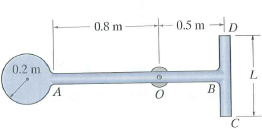Problem

# The pendulum consists of a disk having a mass of 6 kg and slender rods AB and DC which hav...

The pendulum consists of a disk having a mass of 6 kg and slender rods AB and DC which have a mass of 2 kg/m. Determine the length L of DC so that the center of the mass is at the bearing O. What is the moment of inertia of the assembly about an axis perpendicular to the page and passing through point O?#### Step-by-Step Solution

Solution 1

Dolution:

Measured from the right side,

\begin{aligned} &\bar{y}=\frac{6(1.5)+2(1.3)(0.65)}{6+1.3(2)+L(2)}=0.5 \\ &L=6.39 \mathrm{~m} \\ &I_{0}=\frac{1}{2}(6)(0.2)^{2}+66(1)^{2}+\frac{1}{12}(2)(1.3)(1.3)^{2} \\ &\quad+\frac{1}{12}(2)(6.39)(6.39)^{2}+2(6.39)(0.5)^{2} \\ &I_{0}=53.2 \mathrm{~kg} \cdot \mathrm{m}^{2} \end{aligned}